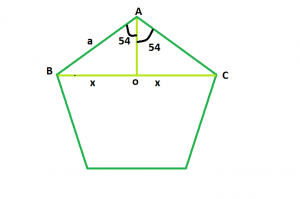# Diagonal of a Regular Pentagon

Given an integer a which is the side of a regular pentagon, the task is to find and print the length of its diagonal.Examples:

Input: a = 6
Output: 7.32
Input: a = 9
Output: 10.98

Approach: We know that the sum of interior angles of a polygon = (n – 2) * 180 where, n is the no. of sides in the polygon.
So, sum of interior angles of pentagon = 3 * 180 = 540 and each interior angle will be 108
Now, we have to find BC = 2 * x. If we draw a perpendicular AO on BC, we will see that the perpendicular bisects BC in BO and OC, as triangles AOB and AOC are congruent to each other.
So, in triangle AOB, sin(54) = x / a i.e. x = 0.61 * a
Therefore, diagonal length will be 2 * x i.e. 1.22 * a.
Below is the implementation of the above approach:

## C++

 `// C++ Program to find the diagonal` `// of a regular pentagon` `#include ` `using` `namespace` `std;`   `// Function to find the diagonal ` `// of a regular pentagon` `float` `pentdiagonal(``float` `a)` `{`   `    ``// Side cannot be negative` `    ``if` `(a < 0)` `        ``return` `-1;`   `    ``// Length of the diagonal` `    ``float` `d = 1.22 * a;` `    ``return` `d;` `}`   `// Driver code` `int` `main()` `{` `    ``float` `a = 6;` `    ``cout << pentdiagonal(a) << endl;` `    ``return` `0;` `}`

## Java

 `// Java Program to find the diagonal` `// of a regular pentagon` `class` `GFG` `{` `    `  `// Function to find the diagonal ` `// of a regular pentagon` `static` `double` `pentdiagonal(``double` `a)` `{`   `    ``// Side cannot be negative` `    ``if` `(a < ``0``)` `        ``return` `-``1``;`   `    ``// Length of the diagonal` `    ``double` `d = ``1.22` `* a;` `    ``return` `d;` `}`   `// Driver code` `static` `public` `void` `main (String args[])` `{` `    ``double` `a = ``6``;` `    ``System.out.println(pentdiagonal(a));` `}` `}`   `// This code is contributed` `// by Akanksha Rai`

## Python3

 `# Python3 Program to find the diagonal ` `# of a regular pentagon `   `# Function to find the diagonal ` `# of a regular pentagon ` `def` `pentdiagonal(a) : `   `    ``# Side cannot be negative ` `    ``if` `(a < ``0``) : ` `        ``return` `-``1`   `    ``# Length of the diagonal ` `    ``d ``=` `1.22` `*` `a ` `    ``return` `d`   `# Driver code ` `if` `__name__ ``=``=` `"__main__"` `:`   `    ``a ``=` `6` `    ``print``(pentdiagonal(a)) `   `# This code is contributed by Ryuga`

## C#

 `// C# Program to find the diagonal` `// of a regular pentagon` `using` `System;`   `public` `class` `GFG{` `    `  `// Function to find the diagonal ` `// of a regular pentagon` `static` `double` `pentdiagonal(``double` `a)` `{`   `    ``// Side cannot be negative` `    ``if` `(a < 0)` `        ``return` `-1;`   `    ``// Length of the diagonal` `    ``double` `d = 1.22 * a;` `    ``return` `d;` `}`   `// Driver code` `    ``static` `public` `void` `Main (){` `    ``double` `a = 6;` `    ``Console.WriteLine(pentdiagonal(a));` `    ``}` `}`

## PHP

 ``

## Javascript

 ``

Output:

`7.32`

Time Complexity: O(1)

Auxiliary Space: O(1)

Feeling lost in the world of random DSA topics, wasting time without progress? It's time for a change! Join our DSA course, where we'll guide you on an exciting journey to master DSA efficiently and on schedule.
Ready to dive in? Explore our Free Demo Content and join our DSA course, trusted by over 100,000 geeks!

Previous
Next